What is a Neural Network?

# What is a Neural Network?Before we get started with the process of building a Neural Network, we need to understand first what a Neural Network is.

A neural network is a collection of neurons connected by synapses. This collection is organized into three main layers: the input layer, the hidden layer, and the output layer.

In an artificial neural network, there are several inputs, which are called features, producing a single output, known as a label.

#### Analogy between Human Mind and Neural Network

Scientists believe that a living creature’s brain processes information through the use of a biological neural network. The human brain has as many as 100 trillion synapses – gaps between neurons – which form specific patterns when activated.

In the field of Deep Learning, a neural network is represented by a series of layers that work much like a living brain’s synapses. It is becoming a popular course now, with an array of career opportunities. Thus, Deep learning Certification in Gurgaon is a must for everyone.

Scientists use neural networks to teach computers how to do things for themselves. The whole concept of Neural network and its varied applications are pretty interesting. Moreover, with the matchless Neural Networks Training in Delhi, you need not look any further.

There are numerous kinds of deep learning and neural networks:

1. Feedforward Neural Network – Artificial Neuron
2. Radial basis function Neural Network
3. Kohonen Self Organizing Neural Network
4. Recurrent Neural Network (RNN) – Long Short Term Memory
5. Convolutional Neural Network
6. Modular Neural Network#### Working of a Simple Feedforward Neural Network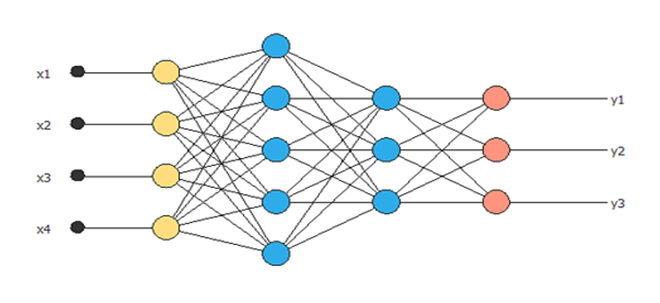1. It takes inputs as a matrix (2D array of numbers).
2. Multiplies the input by a set weight (performs a dot product aka matrix multiplication).
3. Applies an activation function.
4. Returns an output.
5. Error is calculated by taking the difference from the desired output from the data and the predicted output. This creates our gradient descent, which we can use to alter the weights.
6. The weights are then altered slightly according to the error.
7. To train, this process is repeated 1,000+ times. The more the data is trained upon, the more accurate our outputs will be.

#### Implementation of a Neural Network with Python and Keras

Keras has two types of models:

• Sequential model
• The model class used with functional API

Sequential model is probably the most used feature of Keras. Primarily, it represents the array of Keras Layers. It is convenient and builds different types of Neural Networks really quick, just by adding layers to it. Keras also has different types of Layers like Dense Layers, Convolutional Layers, Pooling Layers, Activation Layers, Dropout Layers etc.

The most basic layer is Dense Layer. It has many options for setting the inputs, activation function and so on. So, let’s see how one can build a Neural Network using Sequential and Dense.

First, let’s import the necessary code from Keras: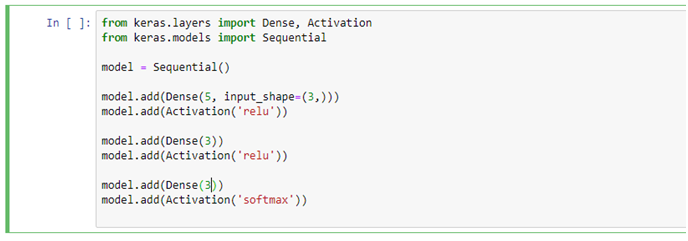After this step, the model is ready for compilation. The compilation step asks to define the loss function and the kind of optimizer which should be used. These options depend on the problem one is trying to solve.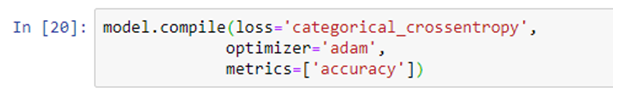Now, the model is ready to get trained. Thus, the parameters get tuned to provide the correct outputs for a given input. This can be done by feeding inputs at the input layer and then, getting an output.

After this one can calculate the loss function using the output and use backpropagation to tune the model parameters. This will fit the model parameters to the data.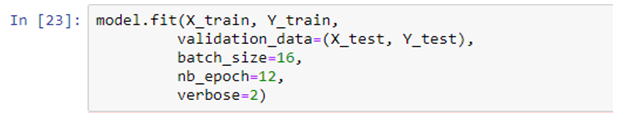Output of the above cell:-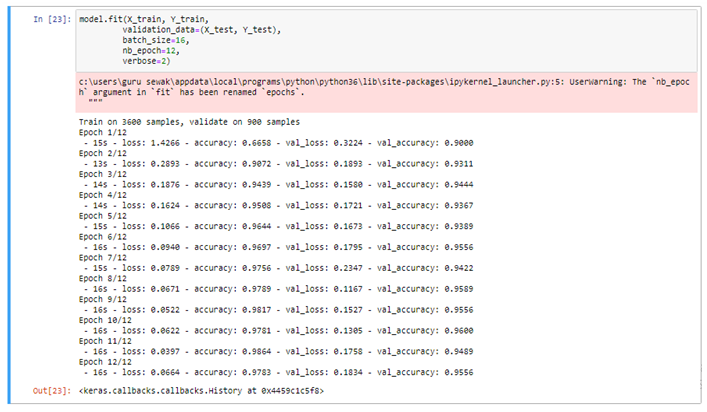This output shows the loss decrease and the accuracy increase over time. At this point, one can experiment with the hyper-parameters and neural network architecture to get the best accuracy.

After getting the final model architecture, one can now take the model and use feed-forward passes and predict inputs. To start making predictions, one can use the testing dataset in the model that has been created previously. Keras enables one to make predictions by using the .predict() function.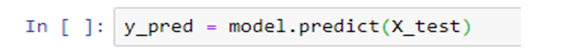#### Some points to be remembered while building a strong Neural Network

1. Adding Regularization to Fight Over-Fitting

The predictive models mentioned above are prone to a problem of overfitting. This is a scenario whereby the model memorizes the results in the training set and isn’t able to generalize on data that it hasn’t seen.

In neural networks, regularization is the technique that fights overfitting by adding a layer in the neural network. It can be done in 3 ways:

• L1 Regularization
• L2 Regularization
• Dropout Regularization

Out of these, Dropout is a commonly used regularization technique. In every iteration, it adds a Dropout layer in the neural network and thereby, deactivates some neurons. The process of deactivating neurons is usually random.

2. Hyperparameter Tuning

Grid search is a technique that you can use to experiment with different model parameters to obtain the ones that give you the best accuracy. This is done by trying different parameters and returning those that give the best results. It helps in improving model accuracy.

#### Conclusion

Neural Network is coping with the fast pace of the technology of the age remarkably well and thereby, inducing the necessity of courses like Neural Network Machine Learning PythonNeural Networks in Python course and more. Though these advanced technologies are just at their nascent stage, they are promising enough to lead the way to the future.

In this article, Building and Training our Neural Network is shown. This simple Neural Network can be extended to Convolutional Neural Network and Recurrent Neural Network for more advanced applications in Computer Vision and Natural Language Processing respectively.

Reference Blogs:

https://keras.rstudio.com